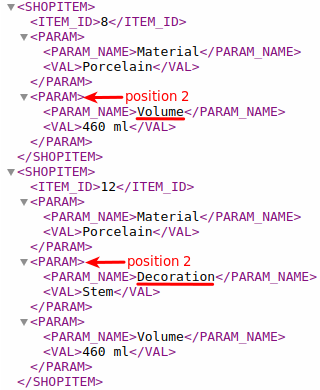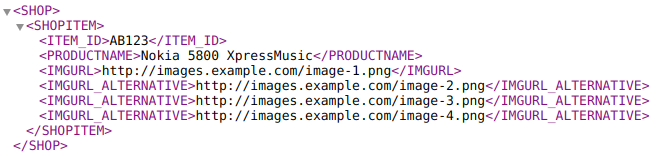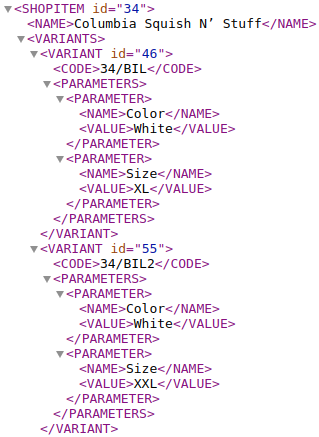# @@POSITION

In this post, we will learn:

## Introduction

When targeting element values, it is sometimes necessary to specify the exact position of the element value, typically for multi-value elements. The special attribute @@POSITION will help us when writing the path to the element.

## What is @@POSITION and what is it for?

The @@POSITION is one of the special attributes that we can work with in the MERGADO Editor, which are part of the Element-Path language. It is used in conditions in element-paths, where it corresponds to the position of the value of the element to which it binds, and is thus used to target a given element position (or multiple positions).

Each element value (even an empty value) has a precise position that can be quantified by natural number (so the position cannot be negative or zero, only 1, 2, 3, …).

Example:

``````<ITEM_ID>8</ITEM_ID>                                        @@POSITION = 1
<EAN>4028145079671</EAN>                                    @@POSITION = 1
<URL>https://www.url.com</URL>                              @@POSITION = 1
<PRICE_VAT>249</PRICE_VAT>                                  @@POSITION = 1
<PARAM>                                                     @@POSITION = 1
<PARAM_NAME>Material</PARAM_NAME>                       @@POSITION = 1
<VAL>Porcelain</VAL>                                     @@POSITION = 1
</PARAM>
<PARAM>                                                     @@POSITION = 2
<PARAM_NAME>Volume</PARAM_NAME>                          @@POSITION = 1
<VAL>460 ml</VAL>                                       @@POSITION = 1
</PARAM>
<PRODUCT>Mug Science Medicine</PRODUCT>                   @@POSITION = 1
<IMAGES>                                                    @@POSITION = 1
<IMAGE>url 1</IMAGE>                                    @@POSITION = 1
<IMAGE>url 2</IMAGE>                                    @@POSITION = 2
<IMAGE>url 3</IMAGE>                                    @@POSITION = 3
<IMAGE>url 4</IMAGE>                                    @@POSITION = 4
</IMAGES>
``````

Example path: `IMAGES | IMAGE { @@POSITION = 3 }`
Read: take the IMAGES element and target its child IMAGE with a value in the third position.

Note that most values have a position of 1. Only multi-value elements (in the example, the PARAM and IMAGE elements) can have a position greater than 1.

## Usage

### General

The special attribute @@POSITION is mainly used when working with multi-value elements, since for simple elements (elements that are not multi-value) when writing the path to the element value it is not necessary to specify the position, since it is always equal to 1 for these elements.

Example of a simple element:

``````<ITEM_ID>8</ITEM_ID>                                        @@POSITION = 1
``````

The `ITEM_ID` path targets the same value as the `ITEM_ID { @@POSITION = 1 }` path.MERGADO 1 did not work with nested or multi-value elements, so the special @@POSITION attribute could not be used in MERGADO 1 either.

At the same time, the weakness of @@POSITION is that it only refers to a position and does not address what value is at that position. For example, if we refer to position 2, where we expect the parameter Volume, it may happen that for some products we actually target the parameter Decoration, because different products may have parameters with different order.If we want to target only the values of the Volume parameter, the path will look like:
`PARAM { PARAM_NAME = "Volume" } | VAL`

### Examples

1. Images: e.g. IMGURL_ALTERNATIVE. Consider the following data:Let’s say we want to target only `image-3.png` and `image-4.png` in the rule. The path will look like this: `IMGURL_ALTERNATIVE { @@POSITION >= 2 }`.

2. Variants: e.g. `VARIANTS | VARIANT`. Consider the following data:Let’s say for the second variant we want to rewrite its size XXL to S. In the rewrite rule, we write the path `VARIANTS | VARIANT { @@POSITION = 2 } | PARAMETERS | PARAMETER { NAME = "Size" } | VALUE`. This path will target the value of the Size parameter only for the second variant.

## Summary

• What is @@POSITION and what is it for?
The @@POSITION is one of the special attributes that we can work with in the MERGADO Editor, which are part of the Element-Path language. It is used in conditions in element-paths, where it corresponds to the position of the value of the element to which it binds, and is thus used to target a given element position (or multiple positions).

• When will we use @@POSITION?
• For multi-value elements, since for simple elements (elements that are not multi-value) there is no need when writing the path to the value element, because it is always equal to 1 for these elements.
• The weakness of @@POSITION is that it only refers to the position and does not address what the value at that position is. So let’s be careful when using @@POSITION for parameters, categories, images, variants, etc.